# Chapter 3 Review of properties of vectors A

• Slides: 28Chapter 3 • Review of properties of vectors • A vector is a quantity with Magnitude and Direction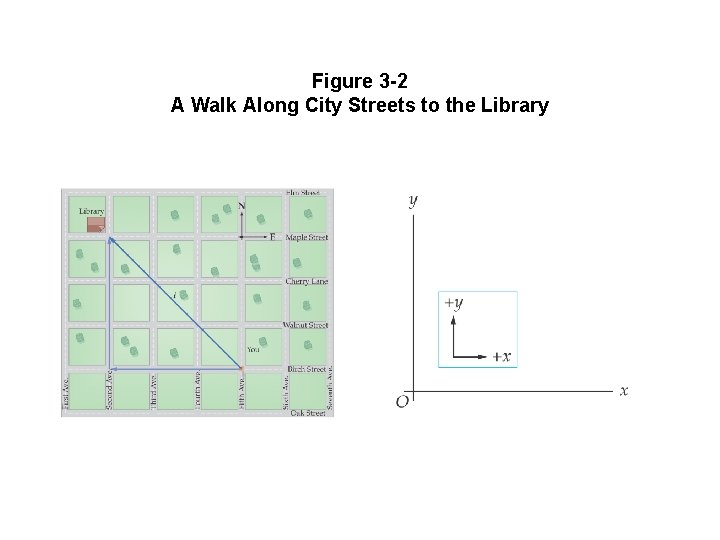Figure 3 -2 A Walk Along City Streets to the Library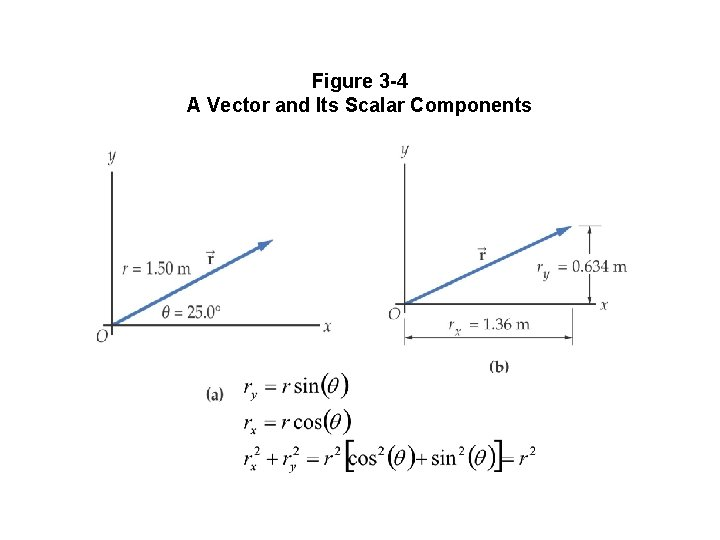Figure 3 -4 A Vector and Its Scalar ComponentsExample 3 -1 Determining the Height of a Cliff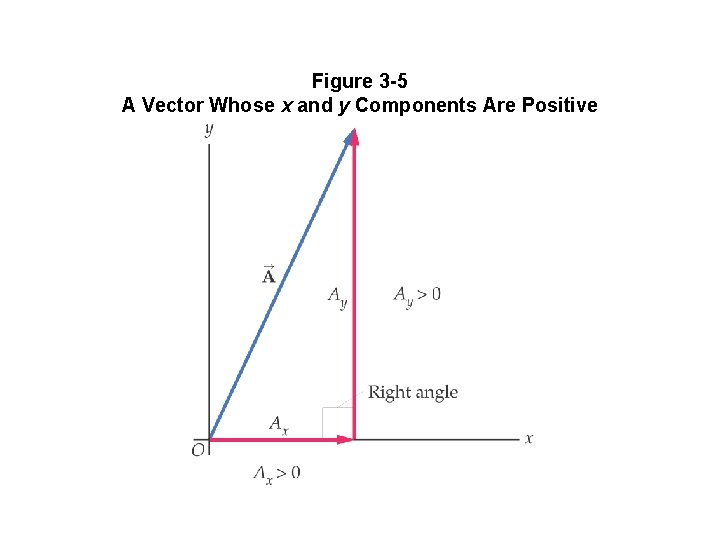Figure 3 -5 A Vector Whose x and y Components Are PositiveFigure 3 -6 ab Examples of Vectors with Components of Different Signs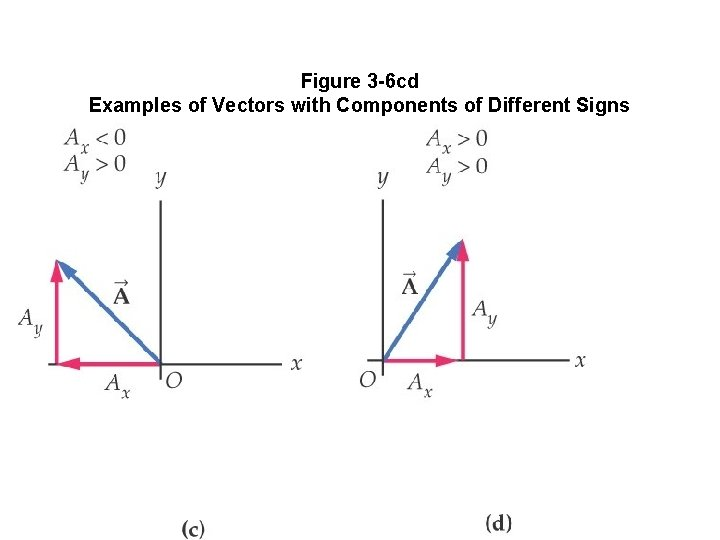Figure 3 -6 cd Examples of Vectors with Components of Different SignsFigure 3 -7 Vector Angle Most often, the angle to the “x” axis will be given.Figure 3 -8 The Sum of Two Vectors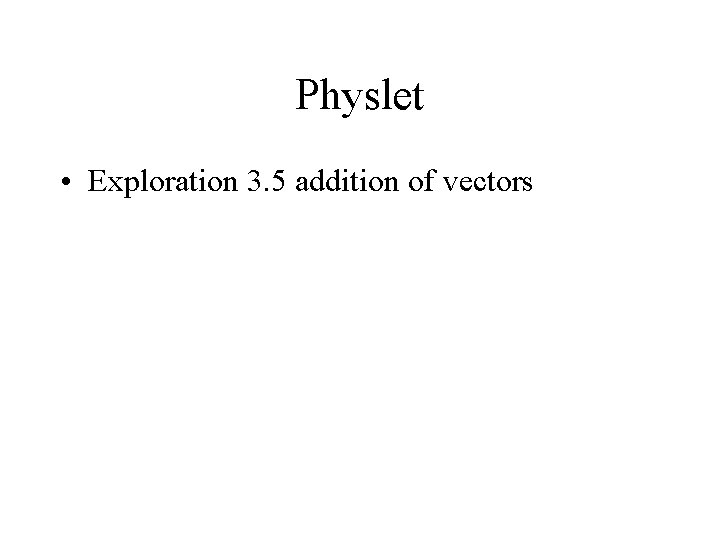Physlet • Exploration 3. 5 addition of vectorsFigure 3 -9 Adding Several VectorsFigure 3 -10 Identical Vectors A at Different Locations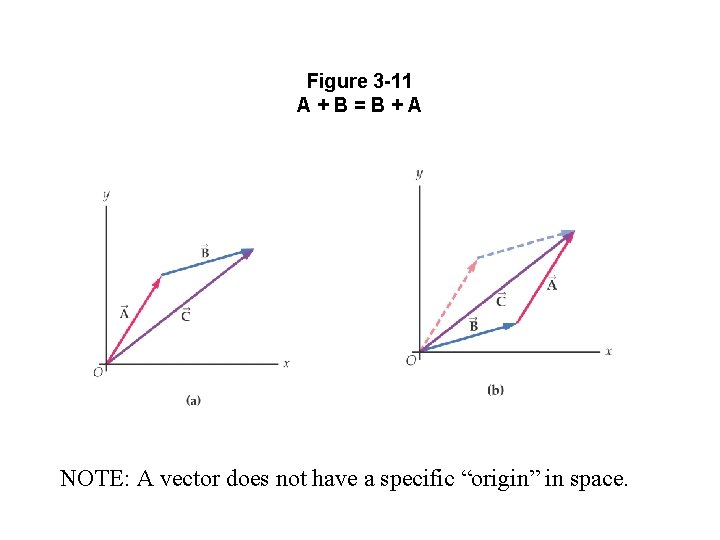Figure 3 -11 A+B=B+A NOTE: A vector does not have a specific “origin” in space.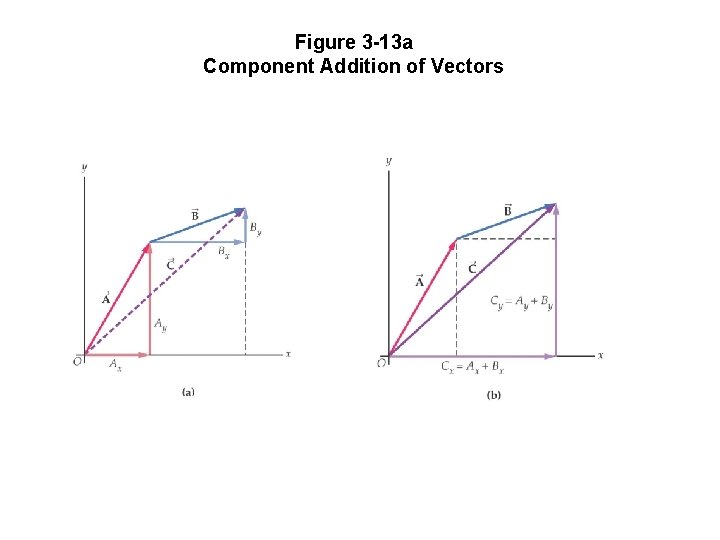Figure 3 -13 a Component Addition of Vectors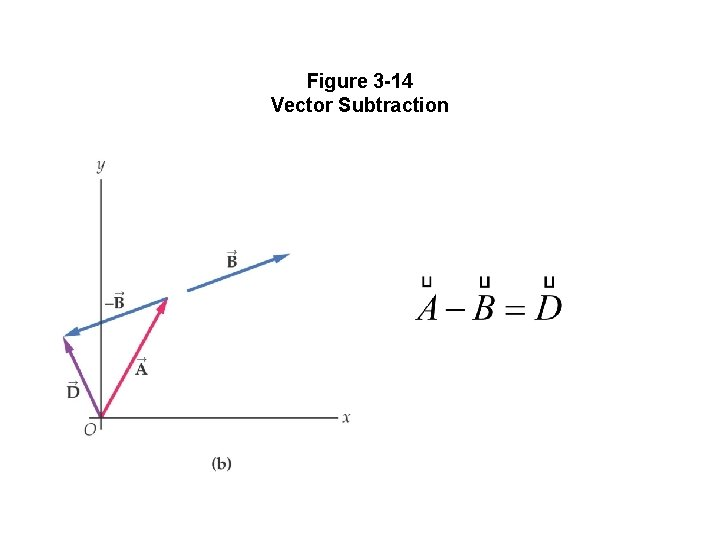Figure 3 -14 Vector Subtraction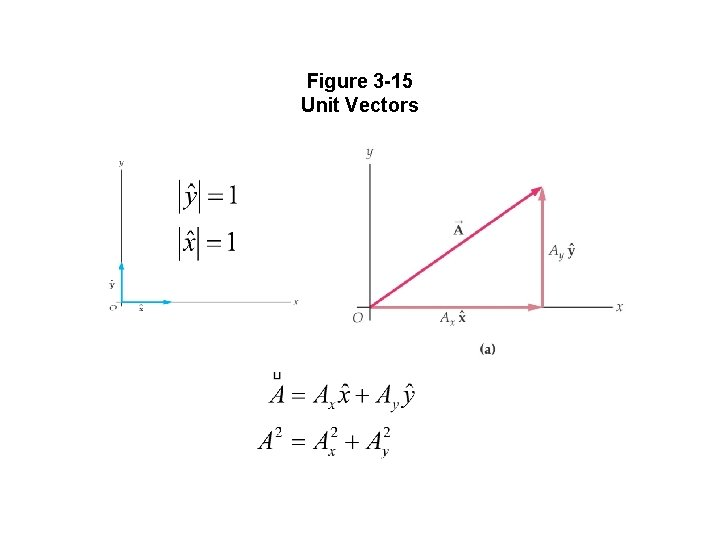Figure 3 -15 Unit Vectors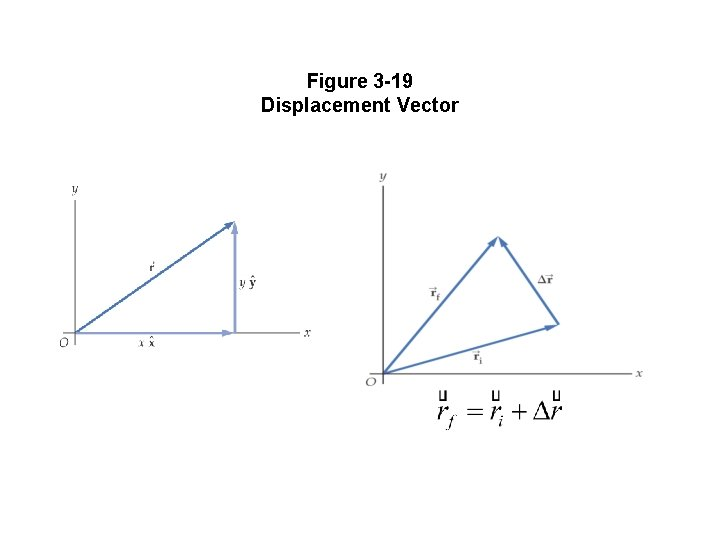Figure 3 -19 Displacement Vector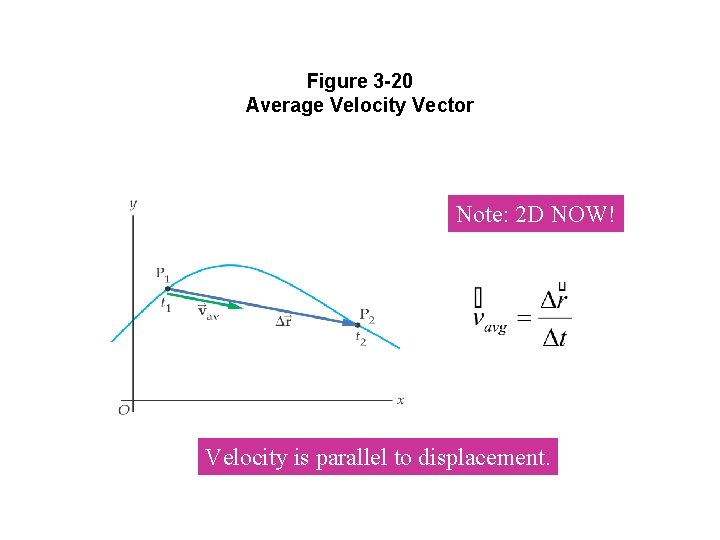Figure 3 -20 Average Velocity Vector Note: 2 D NOW! Velocity is parallel to displacement.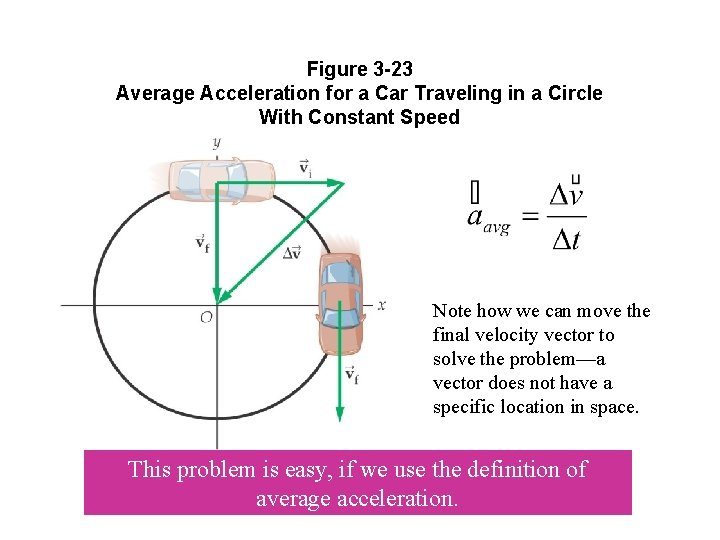Figure 3 -23 Average Acceleration for a Car Traveling in a Circle With Constant Speed Note how we can move the final velocity vector to solve the problem—a vector does not have a specific location in space. This problem is easy, if we use the definition of average acceleration.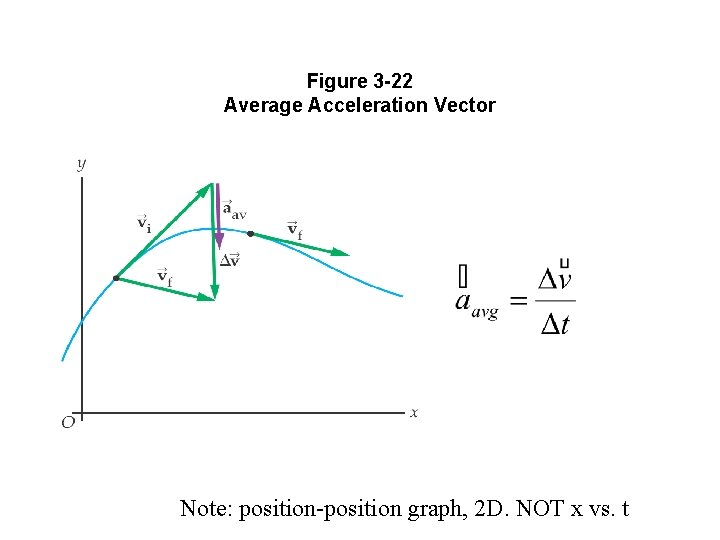Figure 3 -22 Average Acceleration Vector Note: position-position graph, 2 D. NOT x vs. tFigure 3 -24 Velocity and Acceleration Vectors for a Particle Moving Along a Winding Path How do we know what direction the acceleration points? Note: this is a “distance-distance” graph, not distance-time.Figure 3 -25 Relative Velocity of a Passenger on a Train with Respect to a Person on the Ground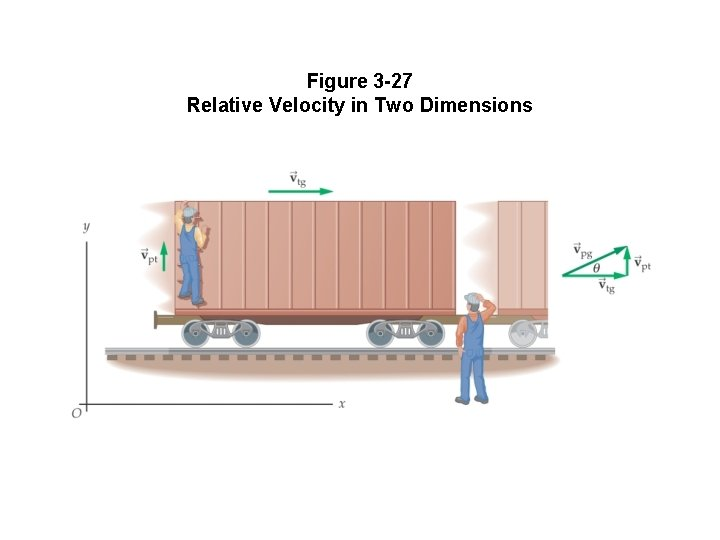Figure 3 -27 Relative Velocity in Two DimensionsExample 3 -2 Crossing a River Vheading Vtrack Navigation Problem: Given desired track and speed of water, what heading should you take? Vwater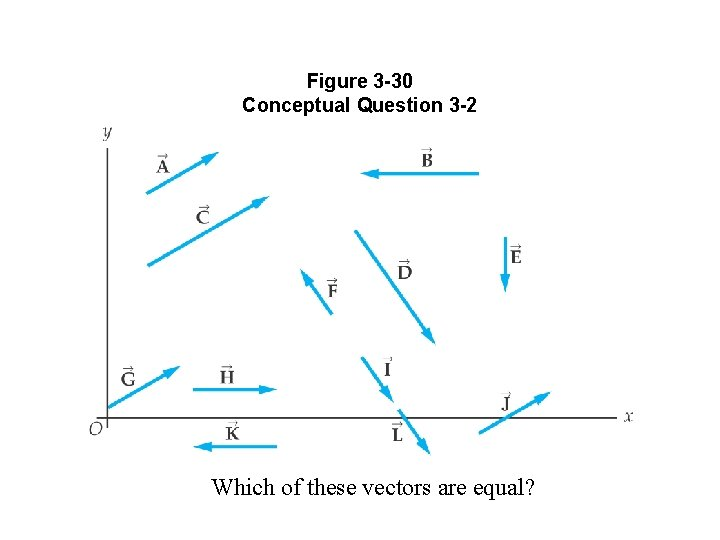Figure 3 -30 Conceptual Question 3 -2 Which of these vectors are equal?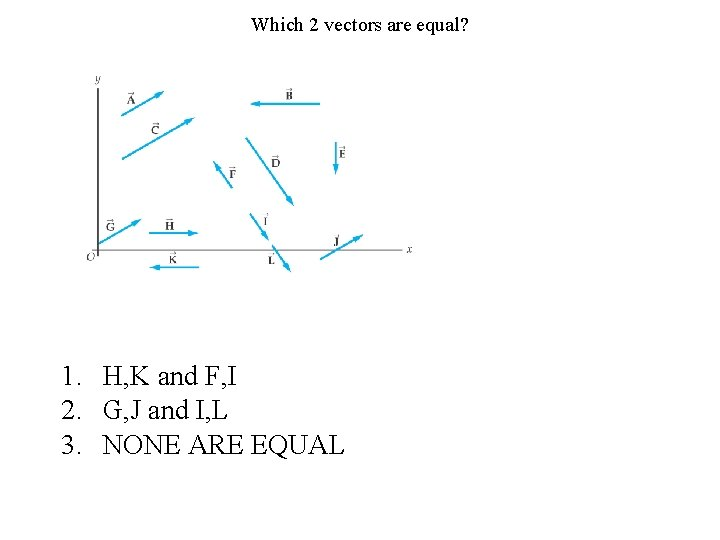Which 2 vectors are equal? 1. H, K and F, I 2. G, J and I, L 3. NONE ARE EQUALFigure 3 -36 Problems 3 -28 and 3 -29Figure 3 -38 Problem 3 -53